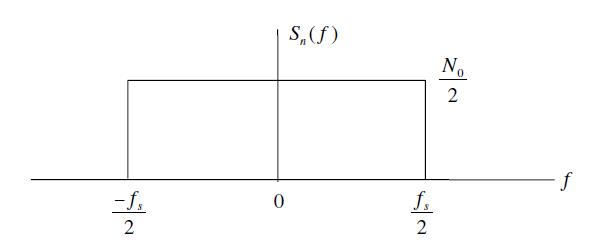# Wireless Channel Modeling: Back to Fundamentals

When a wireless signal travels from a transmitter (Tx) to a receiver (Rx) it undergoes some changes. In simple terms the signal s(t) is scaled by a factor h(t) and noise n(t) is added at the receiver. Let’s take this discussion forward with a simple example. Suppose the Tx transmits one of two possible symbols, +1 or -1. In technical lingo this is called Binary Phase Shift Keying (BPSK). If the channel scaling factor is 0.1 we will either get a +0.1 or -0.1 at the Rx to which AWGN noise is added. The noise is random in nature (having a Gaussian distribution) but for simplicity we assume that it can have one of two values, +0.01 or -0.01.

Continue reading Wireless Channel Modeling: Back to Fundamentals

# Noise Calibration in Simulation of Communication Systems

We have been using a wireless signal model in our simulations without going into the details of noise calibration for simulation. In this article we discuss this. Lets assume the received signal is given as

r(t)=s(t)+n(t)

where r(t) is the received signal s(t) is the transmitted signal and n(t) is the Additive White Gaussian Noise (AWGN). Channel fading is ignored at the moment. Signal to noise ratio for simulation of digital communication systems is given as

ρ=Eb/No (1)

Where Eb is the energy per bit and No is the noise Power Spectral Density (PSD). We also know that for the case of Additive White Gaussian Noise the noise power is given as [Tranter]

σ^2=(No/2)*fs

No=2*(σ^2)/fsWhere σ is the standard deviation of noise and fs is the sampling frequency.  Substituting in equation 1 we get

ρ=Eb/No=Eb/(2*σ^2/fs )

Eb/No=(Eb*fs)/(2*σ^2)

σ^2=(Eb*fs)/(2*Eb/No )

σ=√((Eb*fs)/(2*Eb/No ))

If the energy per bit and the sampling frequency is set to 1 the above equation reduces to

σ=√(1/(2*Eb/No ))

The simulation software can thus calculate the noise standard deviation (or variance) for each value of Eb/No in the simulation cycle. The following piece of MATLAB code generates AWGN with the required power and adds it to the transmitted signal.

```s=sign(rand-0.5);      % Generate a symbol
sigma=1/sqrt(2*EbNo);  % Calculate noise standard deviation
n=sigma*randn;         % Generate AWGN with the required std dev
r=s+n;                 % Add noise to the signal```

How can we assume that energy per bit and sampling frequency is equal to one and are we breaking some discrete time signal processing rule here. This will be discussed in a later post.

# Detecting Sinusoids in Noise

We have previously discussed the problem of detecting two closely spaced sinusoids using the Discrete Fourier Transform (DFT). We assumed that the data set we got was pure i.e. there was no noise. However, in reality this is seldom the case. There is always some noise, corrupting the signal. Let us now see how it effects the detection problem.

We consider Additive White Gaussian Noise (AWGN) as the corrupting source. The noise power is set equal to the power of the two sinusoids i.e. we have an SNR of 0 dB. This is quite a severe case, the noise power is usually a few dB below the signal power. We are also bounded by the number of samples, N=64, giving us a resolution of 15.87 Hz.

```clear all
close all

fm1=100;
fm2=120;
fs=1000;
Ts=1/fs;
N=64;
t=0:Ts:(N-1)*Ts;

x1=sqrt(2)*cos(2*pi*fm1*t);
x2=sqrt(2)*cos(2*pi*fm2*t);
wn=randn(1,N);
x=x1+x2+wn;
W=exp(-j*2*pi/N);

n=0:N-1;
k=0:N-1;

X=x*(W.^(n'*k));

plot(k/N,20*log10(abs(X)))
xlabel ('Normalized Frequency')
ylabel ('X(dB)')```

The data is plotted on a logarithmic scale so that we can compare the signal and noise  power levels.